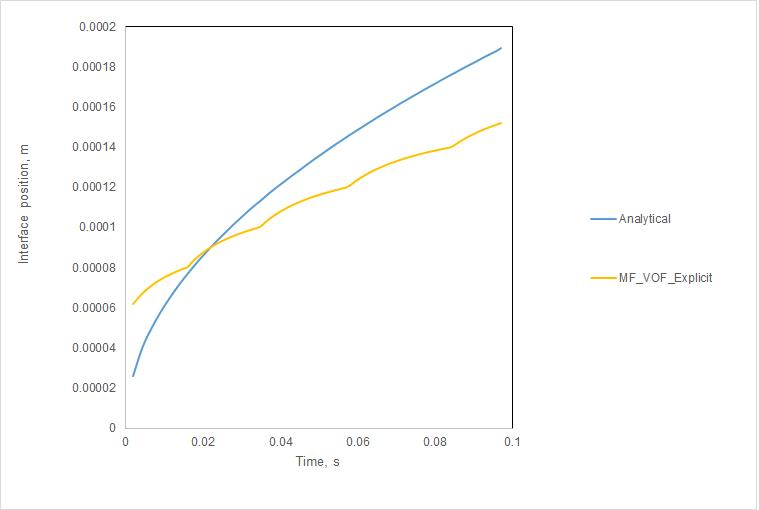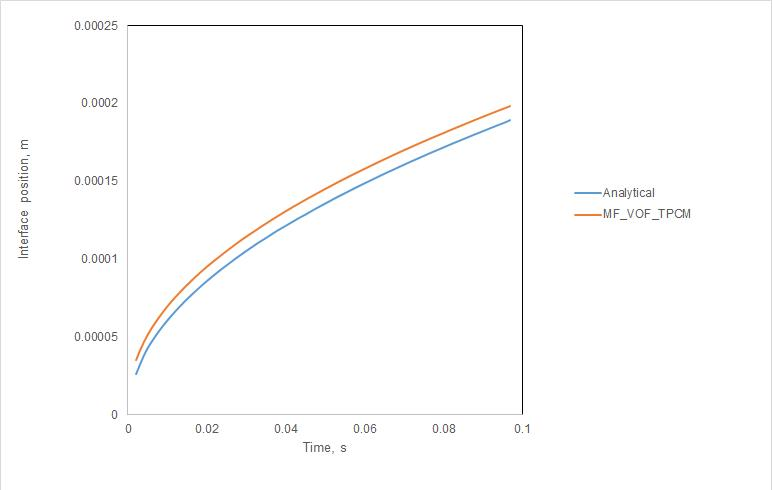# How to combine Multi-Fluid VOF with Geo-Reconstruct and energy source in a phase change process?

Hi,

my goal is to solve 1D Stefan problem on a mesh consisted of 50 cells (3 cells of superheated water-vapor adjacent to 10 K superheated wall and the rest is saturated water-liquid; linear temperature profile in the vapor cells) using Multi-fluid VOF model (sharp interface modeling) available in ANSYS Fluent 2019 R3. The most promising results is obtained with explicit VOF formulation, but the problem is that the slope of interface (alpha_g = 0.5) evolution is not in a good agreement with the analytical solution as shown in the attached figure.

The mass transfer is defined in accordance to Mr. Amine's recommendation (ttps://studentcommunity.ansys.com/thread/zone-of-applicability--define_mass_transfer/?order=all#comment-7a51709b-3091-4657-8157-aa4d00d4cf4d) in a sense that involves volume fraction gradient and reads

where "Kg" is thermal conductivity of vapor, "grad(T_g)" is temperature gradient of the vapor, "grad(alpha_g)" is gradient of vapor volume fraction and "h_lg" is the latent heat.

This equation is included in "DEFINE_MASS_TRANSFER" macro, while the gradient multiplication is realized using UDM inside "DEFINE_ADJUST" macro.

Also, the energy equation of each phase is supplemented with necessary energy source term (S_e.v = m_lg * h_lg; S_e.l = - S_e.v).

Together with the aforementioned mass transfer model, the available phase interaction terms are used: drag ("anisotropic-drag"), heat (Nu = 1) and interfacial area ("ia-gradient").

The applied discretisation schemes are as follows: Phase Coupled SIMPLE (pressure-velocity coupling), Least Squares Cell Based (gradient), PRESTO! (pressure), First Order Upwind (momentum and energy), Geo-Reconstruct (volume fraction) and First Order Implicit (transient).

I tried with other interface reconstruction approaches, but Geo-Reconstruct produced the best result.

Finally, my question is: "How can I improve the solution process in order to have smooth distribution of the interface position?"

Alen• edited January 31
Thermal phase model with temperature dependent nusselt correlations can be tried. Your transfer rate does not do side the liquid side. It is incomplete.
• edited February 3

Dear Mr. Amine,

thank you very much on your help!

The result is much better now, as shown in the attached figure.

Here, "Thermal Phase Change" mass transfer model is used together with "Two-Resistance" interfacial heat transfer model. The latter is supplemented with "Zero-Resistance" model (from phase) and "Ranz-Marshall" model (to phase).

Regarding your comment on the gradient in the liquid side in the mass transfer equation, it is neglected due to existence of liquid at saturation temperature. Therefore, the liquid temperature gradient is zero. For further discussion on this topic, the interested reader is directed to the paper of Sun et al. .

Kind regards,

Alen

P.S.
The physical properties (density, specific heat, thermal conductivity and viscosity) of water-liquid and water-vapor are taken from . Also, the analytical solution shown here is extracted from  using .

References
 D.-L. Sun, J.-L. Xu, L. Wang, Development of a vapor-liquid phase change model for volume-of-fluid method in FLUENT, International Communications in Heat and Mass Transfer, 39, 2012, 1101-1106.
 Y. Sato, B. Ni?eno, A sharp-interface phase change model for a mass-conservative interface tracking method, Journal of Computational Physics, 249, 2013, 127-161.
 A. Rohatgi, WebPlotDigitizer, Version 4.2, 2019.• edited February 3

Nice work. Are you planning to publish: if yes just write a small comment whenever it is online